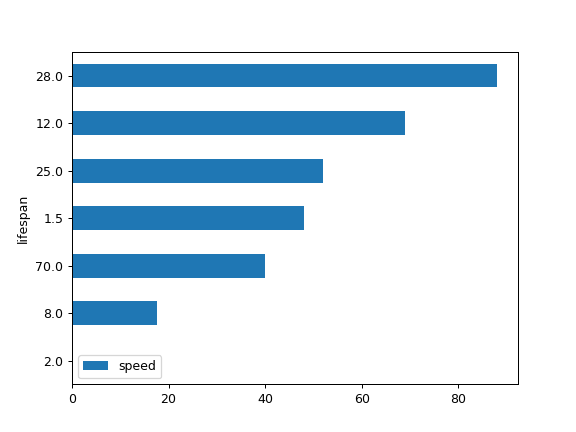# pandas.DataFrame.plot.barh¶

DataFrame.plot.barh(x=None, y=None, **kwargs)[source]

Make a horizontal bar plot.

A horizontal bar plot is a plot that presents quantitative data with rectangular bars with lengths proportional to the values that they represent. A bar plot shows comparisons among discrete categories. One axis of the plot shows the specific categories being compared, and the other axis represents a measured value.

Parameters
xlabel or position, optional

Allows plotting of one column versus another. If not specified, the index of the DataFrame is used.

ylabel or position, optional

Allows plotting of one column versus another. If not specified, all numerical columns are used.

colorstr, array_like, or dict, optional

The color for each of the DataFrame’s columns. Possible values are:

• A single color string referred to by name, RGB or RGBA code,

for instance ‘red’ or ‘#a98d19’.

• A sequence of color strings referred to by name, RGB or RGBA

code, which will be used for each column recursively. For instance [‘green’,’yellow’] each column’s bar will be filled in green or yellow, alternatively.

• A dict of the form {column namecolor}, so that each column will be

colored accordingly. For example, if your columns are called a and b, then passing {‘a’: ‘green’, ‘b’: ‘red’} will color bars for column a in green and bars for column b in red.

New in version 1.1.0.

**kwargs

Additional keyword arguments are documented in DataFrame.plot().

Returns
matplotlib.axes.Axes or np.ndarray of them

An ndarray is returned with one matplotlib.axes.Axes per column when subplots=True.

DataFrame.plot.bar

Vertical bar plot.

DataFrame.plot

Make plots of DataFrame using matplotlib.

matplotlib.axes.Axes.bar

Plot a vertical bar plot using matplotlib.

Examples

Basic example

>>> df = pd.DataFrame({'lab': ['A', 'B', 'C'], 'val': [10, 30, 20]})
>>> ax = df.plot.barh(x='lab', y='val')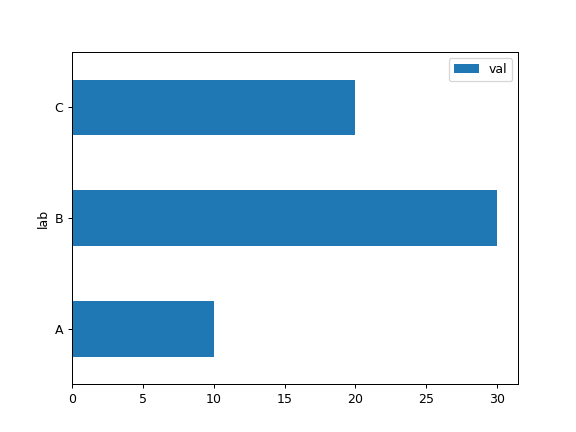Plot a whole DataFrame to a horizontal bar plot

>>> speed = [0.1, 17.5, 40, 48, 52, 69, 88]
>>> lifespan = [2, 8, 70, 1.5, 25, 12, 28]
>>> index = ['snail', 'pig', 'elephant',
...          'rabbit', 'giraffe', 'coyote', 'horse']
>>> df = pd.DataFrame({'speed': speed,
...                    'lifespan': lifespan}, index=index)
>>> ax = df.plot.barh()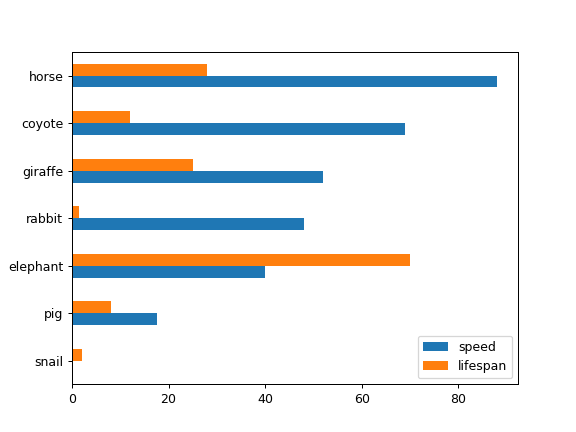Plot stacked barh charts for the DataFrame

>>> ax = df.plot.barh(stacked=True)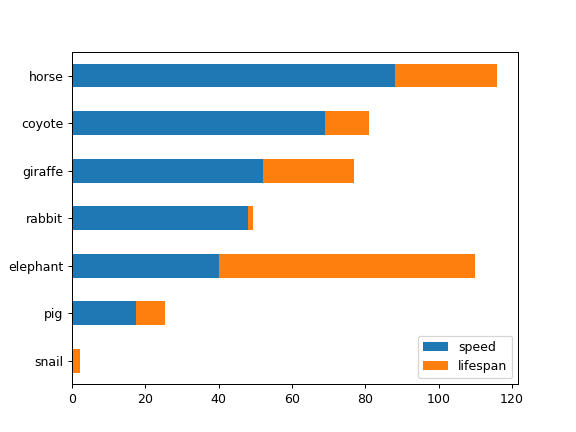We can specify colors for each column

>>> ax = df.plot.barh(color={"speed": "red", "lifespan": "green"})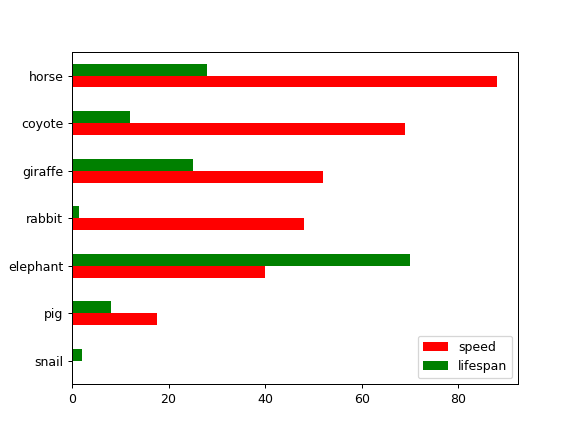Plot a column of the DataFrame to a horizontal bar plot

>>> speed = [0.1, 17.5, 40, 48, 52, 69, 88]
>>> lifespan = [2, 8, 70, 1.5, 25, 12, 28]
>>> index = ['snail', 'pig', 'elephant',
...          'rabbit', 'giraffe', 'coyote', 'horse']
>>> df = pd.DataFrame({'speed': speed,
...                    'lifespan': lifespan}, index=index)
>>> ax = df.plot.barh(y='speed')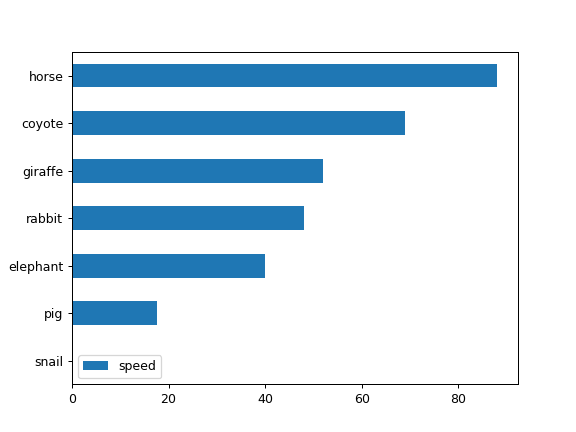Plot DataFrame versus the desired column

>>> speed = [0.1, 17.5, 40, 48, 52, 69, 88]
>>> lifespan = [2, 8, 70, 1.5, 25, 12, 28]
>>> index = ['snail', 'pig', 'elephant',
...          'rabbit', 'giraffe', 'coyote', 'horse']
>>> df = pd.DataFrame({'speed': speed,
...                    'lifespan': lifespan}, index=index)
>>> ax = df.plot.barh(x='lifespan')